### 根号数据结构复习# 根号数据结构复习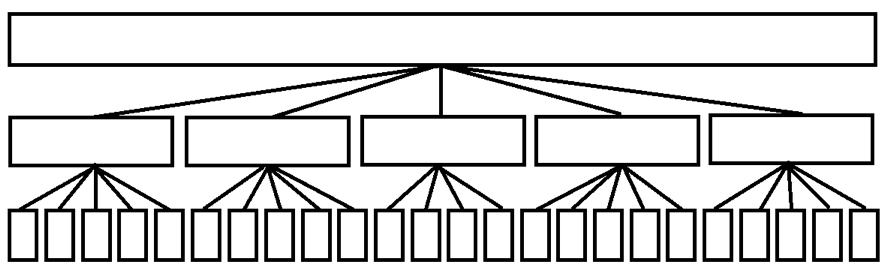## 用根号平衡来优化数据结构复杂度

#### 0x01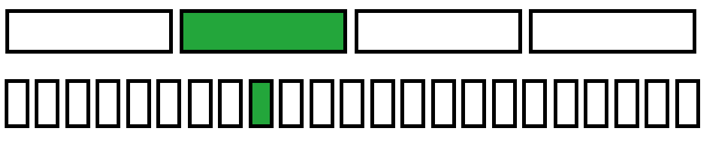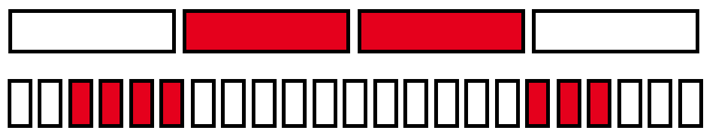#### 0x02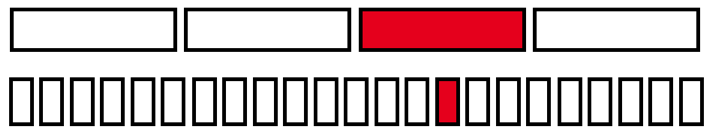#### 0x03#### 0x04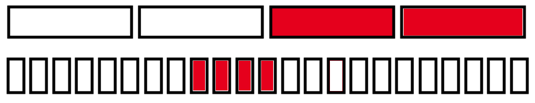#### 0x05#### 0x06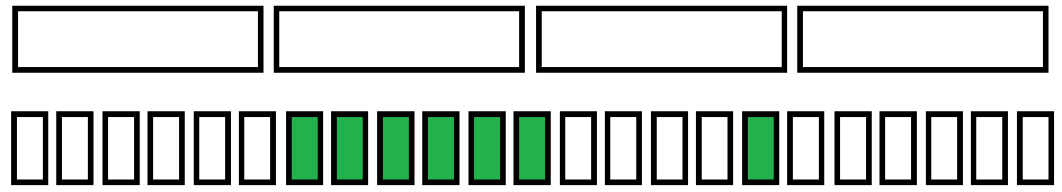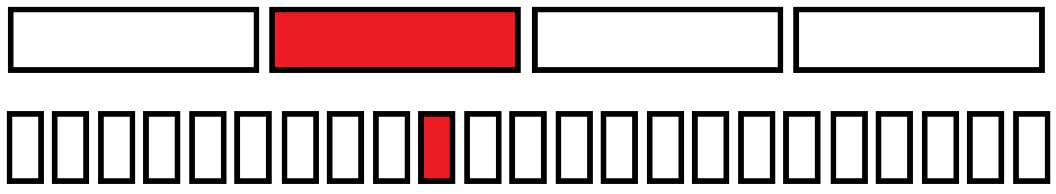#### 0x07 CodeChef Chef and Churu

1. 1 x y 把序列中第 $x$ 个数改成 $y$
2. 2 x y 求第 $x$ 个函数到第 $y$ 个函数的和

## 简单莫队算法

#### 常见优化

• 奇偶块排序，可以快一倍左右。
• 调整块大小 $\left( n / \sqrt { 2m / 3 } \right)$ 可以快 $10\%$ 左右。

#### 0x01 [AHOI2013]作业

$n \leq 10^5,\ m \leq 10^6$。

#### 0x02 [Ynoi2016]这是我自己的发明

• 1 x：将树根换为 $x$
• 2 x y：从 $x$ 的子树中选每一个点，$y$ 的子树中选每一个点，如果两个点点权相等则ans++，求 $ans$。

Solution 1

Solution 2

nzhtl1477 的讲课。